In the circuit shown in figure neglecting source resistance the voltmeter and ammeter reading will respectively, will be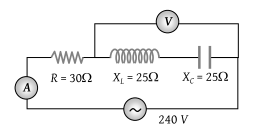(1) 0V, 3A

(2) 150V, 3A

(3) 150V, 6A

(4) 0V, 8A

Concept Questions :-

Different types of AC Circuits
High Yielding Test Series + Question Bank - NEET 2020

Difficulty Level:

In the circuit shown in the figure, the ac source gives a voltage $V=20\mathrm{cos}\left(2000\text{\hspace{0.17em}​}t\right).$ Neglecting source resistance, the voltmeter and ammeter reading will be: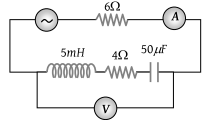(1) 0V, 0.47A

(2) 1.68V, 0.47A

(3) 0V, 1.4 A

(4) 5.6V, 1.4 A

Concept Questions :-

Rms and average values
High Yielding Test Series + Question Bank - NEET 2020

Difficulty Level:

An ac source of angular frequency ω is fed across a resistor r and a capacitor C in series. The current registered is I. If now the frequency of the source is changed to ω/3 (but maintaining the same voltage), the current in the circuit is found to be halved. Calculate the ratio of reactance to resistance at the original frequency ω.

(1) $\sqrt{\frac{3}{5}}$

(2) $\sqrt{\frac{2}{5}}$

(3) $\sqrt{\frac{1}{5}}$

(4) $\sqrt{\frac{4}{5}}$

Concept Questions :-

Different types of AC Circuits
High Yielding Test Series + Question Bank - NEET 2020

Difficulty Level:

For a series RLC circuit R = XL = 2XC. The impedance of the circuit and phase difference (between) V and i will be

(1) $\frac{\sqrt{5}R}{2},\text{\hspace{0.17em}}{\mathrm{tan}}^{-1}\left(2\right)$

(2) $\frac{\sqrt{5}R}{2},{\mathrm{tan}}^{-1}\left(\frac{1}{2}\right)$

(3) $\sqrt{5}{X}_{C},{\mathrm{tan}}^{-1}\left(2\right)$

(4) $\sqrt{5}R,\text{\hspace{0.17em}}{\mathrm{tan}}^{-1}\left(\frac{1}{2}\right)$

Concept Questions :-

Different types of AC Circuits
High Yielding Test Series + Question Bank - NEET 2020

Difficulty Level:

In the adjoining ac circuit the voltmeter whose reading will be zero at resonance is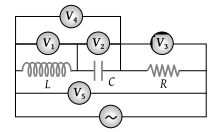(1) V1

(2) V2

(3) V3

(4) V4

High Yielding Test Series + Question Bank - NEET 2020

Difficulty Level:

In the adjoining figure, the impedance of the circuit will be: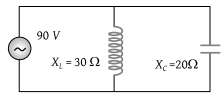(1) 120 ohm

(2) 50 ohm

(3) 60 ohm

(4) 90 ohm

Concept Questions :-

Different types of AC Circuits
High Yielding Test Series + Question Bank - NEET 2020

Difficulty Level:

Which one of the following curves represents the variation of impedance (Z) with frequency f in series LCR circuit

(1)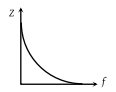(2)(3)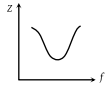(4)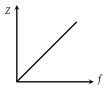High Yielding Test Series + Question Bank - NEET 2020

Difficulty Level:

The variation of the instantaneous current (I) and the instantaneous emf (E) in a circuit is as shown in fig. Which of the following statements is correct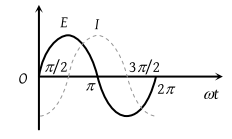(1) The voltage lags behind the current by π / 2

(2) The voltage leads the current by π / 2

(3) The voltage and the current are in phase

(4) The voltage leads the current by π

High Yielding Test Series + Question Bank - NEET 2020

Difficulty Level:

The figure shows the variation of R, XL, and XC with frequency f in a series L, C, R circuit. Then for what frequency point, the circuit is inductive: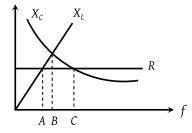(1) A

(2) B

(3) C

(4) All points

Concept Questions :-

Different types of AC Circuits
High Yielding Test Series + Question Bank - NEET 2020

Difficulty Level:

An ac source of variable frequency f is connected to an LCR series circuit. Which one of the graphs in the figure represents the variation of the current I in the circuit with frequency f :

(1)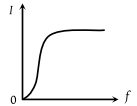(2)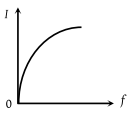(3)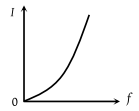(4)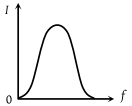Concept Questions :-

Different types of AC Circuits